# What is TensorFrames? TensorFlow + Apache SparkWhat is TensorFrames? TensorFlow + Apache Spark

First thing first, what is TensorFrames?

TensorFrames is an open source created by Apache Spark contributors. Its functions and parameters are named the same as in the TensorFlow framework. Under the hood, it is an Apache Spark DSL (domain-specific language) wrapper for Apache Spark DataFrames. It allows us to manipulate the DataFrames with TensorFlow functionality. And no, it is notpandas DataFrame, it is based on Apache Spark DataFrame.

..but wait, what is TensorFlow (TF)?

TensorFlow is an open-source software library for dataflow and differentiable programming across a range of tasks. It is a symbolic math library and is also used for machine learning applications such as neural networks.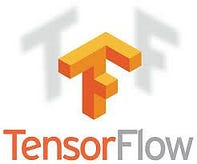..and Apache Spark?

Apache Spark is an open-source distributed general-purpose cluster-computing framework.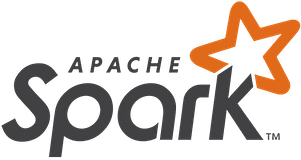A word about scale

Today when we mention scale, we usually talk about two options; scale horizontally, and scaling vertically.

·        Horizontal scale — add additional machines with more or less the same computing power

·        Vertical scale — adding more resources to machine/s we are currently working with. It can be a processor upgraded from a CPU to GPU, more memory (RAM), and etc.

With TensorFrames, we can do both, more processor computing power, and more machines. Where with only TensorFlow we would usually focus on adding more power through scaling vertically, now with Apache Spark support, we can scale both vertically and horizontally. But, how do we know how much of each we actually need? To answer this question, we need to understand the full usage of our applications and plan accordingly.

For each change, like adding a machine or upgrading from CPU to GPU, we have downtime. In the cloud, resizing a cluster or adding more compute power, is a matter of minutes, versus on-prem where we need to deal with adding new machines and upgrading machines processors, this can take days, and sometimes months.

So, A more flexible solution is the public cloud.

In the picture below, scale horizontally is the X-axis where scale vertically is the Y-axis.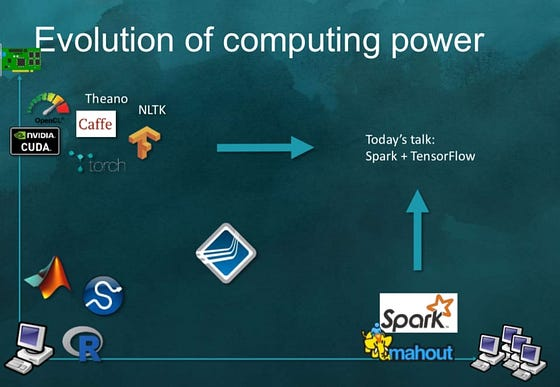**Slide from Tim Hunter presentation at Apache Spark conf

## Tensor

A statically typed multi-dimensional array whose elements are of a generic type.

## GraphDef

`Graph` or `Computional Graph` is the core concept of TensorFlow to present computation. When we use TensorFlow, we first create our own `Computation Graph` and pass the `Graph` to TensorFlow. `GraphDf` is the serialized version of `Graph`.

## Operation

A Graph node that performs computation on Tensors. An Operation is a node in a `Graph` that takes zero or more `Tensors` (produced by other Operations in the Graph) as input and produces zero or more `Tensor` s as output.

## Identity

`tf.identity` is used when we want to explicitly transport tensor between devices (like, from GPU to a CPU). The operation adds nodes to the graph, which makes a copy when the devices of the input and the output are different.

## Constant

A constant has the following arguments which can be tweaked as required to get the desired function. It the same as a variable, but its value can’t be changed. Constant can be:

·        `value`: A constant value (or list) of output type `dtype`.

·        `dtype`: The type of the elements of the resulting tensor.

·        `shape`: Optional dimensions of resulting tensor.

·        `name`: Optional name for the tensor.

·        `verify_shape`: Boolean that enables verification of a shape of values.

## Placeholders

Allocate storage for data (such as for image pixel data during a feed). Initial values are not required (but can be set, see `tf.placeholder_with_default`). Versus variables, where you need to declare the initial value. \

## Dataframe

This is a distributed collection of data organized into named columns that provide operations to filter, group, or compute aggregates. Dataframe data is often distributed across multiple machines. It can be in memory data or on disk.

## RelationalGroupedDataset

A set of methods for aggregations on a `DataFrame`, created by groupBycubeor rollup.

The main method is the `agg` function, which has multiple variants. This class also contains some first-order statistics such as `mean``sum` for convenience.

Now that we understand the terminology better, let’s look at the functionality.

## The Functionality — TensorFlow version 0.6.0

Apache Spark is known for being an analytics platform for data at scale, together with TensorFlow, we get TensorFrames which have three categories of data manipulations:

Let’s understand each functionality.

```-1- Mapping
```

Mapping operations transform and/or adds columns to a given dataframe.

Each functionality is accessed through two API, one which receives Operation and the other which receives DataFrame, GraphDef, and ShapeDescription.

Exposed API:

## MapRows

`def mapRows(o0: Operation, os: Operation*): DataFrame`

For the user, this is the function that will be more often in use, since there is no direct request to create the GraphDef and ShapeDescription object. This way is more readable for experienced TensorFlow developers:

mapRows receives two parameters, operation, and operation* which means the second operation can be a collection of operations. Later it turns them into a sequence and translates it into a graph, it creates the ShapeDiscription out of the graph and sends it with the DataFrame to an internal function. Where it transforms the distributed data row by row according to the transformations given in the graph. All input in the graph should be filled with some data from the given DataFrame or constants. Meaning, we can’t use null. At the end the function returns a new DataFrame with the new schema, the schema will contain the original schema plus new columns that correspond to the graph output. ShapeDiscription provides the shape of the output, it is used, behind the scenes, for optimization and going around limitations of the kernel.

## MapBlock

Performs a similar task as `MapRows`, however, since it is optimized for compact, it applies the graph transformers in blocks of data and not row by row.

`def mapBlocks(o0: Operation, os: Operation*): DataFrame`

The often more used function is:

Code example: We create val df, which is of type DataFrame, with two rows, one contains value 1.0 and the second data row contain value 2.0. The column name is x.

val x is a declaration of the placeholder for the output, y is the identity for transporting tensors from CPU to GPU or from machine to machine, it received val x as it’s value.

z is the computation function itself. Here, `df.MapBlock` functions gets two operations, y and z, and retunes a new DataFrame named df2 with extra column z. Column z is the sum of x+x. In the output, column x is the original value, column y is the identity value and column z is the output of the graph.

## MapBlocksTrimmed

This is the same as `MapBlock` , BUT, it drops the original DataFrame columns from the result DataFrame. Meaning the output DataFrame will contain only the calculated columns.

`def mapBlocksTrimmed(o0: Operation, os: Operation*): DataFrame`

Let’s look at:

Code example: we create a DataFrame named df with two rows with values 3.0 and 4.0 . Notice that we create a constant named out with value 1.0 and 2.0, this constant is the TensorFrame dsl functionality that mimics the TensorFlow functionality. Then we call `df.MapBlocksTrimmed`. The output schema will only contain the result column, which is named "out" and in our case will only hold the constant values which are 1.0 and 2.0 .

Important Note in the first line of code we import TesnorFrames dsl and we name it to tf, which stands for TensorFlow, we do it since this is how TesnorFlow users used to work with it and we are adhering to the best practices of TensorFlow.

```-2- Reducing
```

Reduction operations coalesce a pair or a collection of rows and transform them into a single row, it repeats the same operation until there is one row left. Under the hood, TensorFrames minimizes the data transfer between computers by reducing all the rows on each computer first and then sending the remainder over the network to perform the last reductions.

`f(f(a, b), c) == f(a, f(b, c))`

The transforms function must be classified as morphism: the order in which they are done should not matter. In mathematical terms, given some function f and some function inputs a, b, c, the following must hold: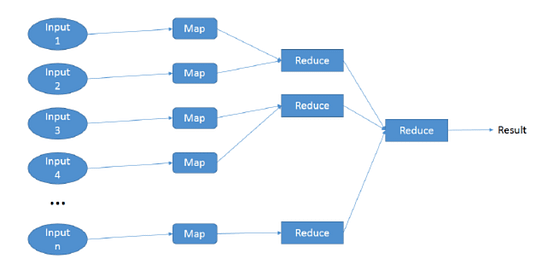Map reduce schema by Christopher Scherb

The reduce functionality API, same as the rest, we have 2 API for each functionality, the one which receives Operation is more intuitive, however, in TensorFlow there is no direct reduce rows operation, instead, there are many reduce operations like `tf.math.reduce_sum` and `tf.reduce_sum` .

## ReduceRows

This functionality uses TensorFlow operations to merge two rows together until there is one row left. It receives the datafram, graph, and a ShapeDescription.

`def reduceRows(o0: Operation, os: Operation*): Row`

User interface:

In the next code example. We create a DataFrame with a column named inand two rows. x1 and x2 placeholder for dtype and x- which is an add operation of x1 and x2. reduceRows, return a Row with value 3 which is the sum of 1.0 and 2.0.

## ReduceBlocks

Works the same as `ReduceRows` , BUT, it does it on a vector of rows and not row by row.

`def reduceBlocks(o0: Operation, os: Operation*): Row`

More used function:

Code example: Here we create a DataFrame with two columns — key2 and x. One placeholder names x1, one reduce_sum TensorFlow operation named x. The reduce functionality return the sum of the rows in the DataFrame according to the desired columns that the reduce_sum named after which is x.

```-3- Aggregation
```

`def aggregate(data: RelationalGroupedDataset, graph: GraphDef, shapeHints: ShapeDescription): DataFrame`

Aggregation is an extra operation for Apache Spark and TensorFlow. It is different from the aggregation functionality in TensorFlow and works with RelationalGroupedDataset. API functionality:

Aggregate receives a RelationalGroupedDataset which is an Apache Spark object, it wraps DataFrame and adds aggregation functionality, a sequence of expressions and a group type.

The aggregate function receives the graph and ShareDescriptiom. It aggregates rows together using reducing transformation on grouped data. This is useful when data is already grouped by key. At the moment, only numerical data is supported.

Code example: In the example, we have a DataFrame with two columns, key, and xx1 as a placeholder and x as the reduce_sum functionality named x.

Using groupby functionality we group the rows by key, and after it, we call aggregate with the operations. We can see in the output that the aggregated was calculated according to the key, for the key with value 1- we received 2.1 as the value for column x and for the key with value 2 we received 2.0 as the value for column x.

#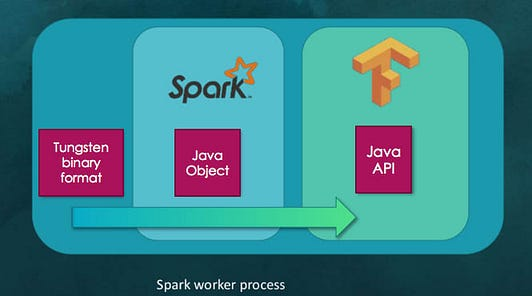In all TensorFrames functionality, the DataFrame is sent together with the computations graph. The DataFrame represents the distributed data, meaning in every machine there is a chunk of the data that will go through the graph operations/ transformations. This will happen in every machine with the relevant data. Tungsten binary format is the actual binary in-memory data that goes through the transformation, first to Apache Spark Java object and from there it is sent to TensorFlow Jave API for graph calculations. This all happens in the Spark Worker process, the Spark worker process can spin many tasks which mean various calculation at the same time over the in-memory data.

# Noteworthy

·        DataFrames with scala is currently an experimental version.

·        The Scala DSL only features a subset of TensorFlow transforms.

·        TensorFrames is open source and can be supported here.

·        Python was the first client language supported by TensorFlow and currently supports the most features. More and more of that functionality is being moved into the core of TensorFlow (implemented in C++) and exposed via a C API. Which later exposed through other languages API, such as Java and JavaScript.

·        Interested in working with Keras? check out Elephas: Distributed Deep Learning with Keras & Spark.

·        interested in TensorFrames project on the public cloud? check this and this.

Now that you know more about TensorFrames, how will you take it forward?

-------------------------------------------------

Thanks for reading :heart: If you liked this post, share it with all of your programming buddies! Follow me on Facebook | Twitter